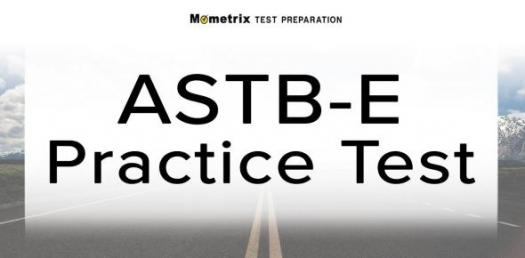# Astb Practice Test

10 Questions | Total Attempts: 1109SettingsThe Aviation Standard Test Battery is an important assessment performed by the United States Navy to select the best brains that will join the service. The performance of an individual in this examination determines if he/she meets the standards for training. This test is in different formats and assesses applicants mathematical, and comprehension abilities as well as aviation knowledge.

• 1.
How many kilometers make one nautical mile?
• A.

2.345 kilometers

• B.

1.852 kilometers

• C.

2.156 kilometers

• D.

2.711 kilometers

• 2.
What enables an aircraft to escape the force of gravity?
• A.

Rudder

• B.

Static lift

• C.

Hot air balloons

• D.

Rotors

• 3.
Which of these is not a criterion for classifying an aircraft?
• A.

Usage

• B.

Brand

• C.

Aircraft propulsion

• D.

Lift type

• 4.
What is the probability of 2019 being a leap year?
• A.

0.25

• B.

0.5

• C.

1

• D.

0.75

• 5.
What part of an aircraft comprises the main load-bearing equipment?
• A.

Structure of the aircraft

• B.

Propulsion system

• C.

Airlift system

• D.

Avionics system

• 6.
Which of these is a prime number?
• A.

6

• B.

10

• C.

5

• D.

12

• 7.
What is the total sum of angles in a triangle?
• A.

270 degrees

• B.

180 degrees

• C.

360 degrees

• D.

240 degrees

• 8.
What is the square root of 144?
• A.

12

• B.

15

• C.

24

• D.

6

• 9.
What does the empennage of an aircraft refer to?
• A.

Tail of the aircraft

• B.

Propeller of the aircraft

• C.

Communication of the aircraft

• D.

• 10.
Which of these operations should be executed first in a math problem?
• A.

Division

• B.

Subtraction

• C.

Multiplication

• D.Back to top